Custom SearchRIGHT TRIANGLES WITH SPECIAL ANGLES AND SIDE RATIOS Three types of right triangles are especially significant because of their frequent occurrence. These are the 30-60-90 triangle, the 45-90 triangle, and the 3-4-5 triangle. THE 30-60-90 TRIANGLE The 30-60-90 triangle is so named because these are the sizes of its three angles. The sides of this triangle are in the ratio of 1 to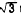to 2, as shown in figure 19- 10.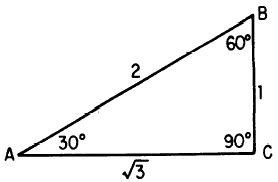Figure 19-lo.-30-60-90" triangle. The sine ratio for the 30 angle in figure 19-10 establishes the proportionate values of the sides. For example, we know that the sine of 30 is 1/2; therefore side AB must be twice as long as BC. If side BC is 1 unit long, then side AB is 2 units long and, by the rule of Pythagoras, AC is found as follows:Regardless of the size of the unit, a 30- 60-90 triangle has a hypotenuse which is 2 times as long as the shortest side. The shortest side is opposite the 30 angle. The side opposite the 60 angle is fl times as long as the shortest side. For example, suppose that the hypotenuse of a 30-60-90 triangle is 30 units long; then the shortest side is 15 units long, and the length of the side opposite the 60 angle is 15units. Practice problems. Without reference to tables or to the rule of Pythagoras, find the following lengths and angles in figure 19-11: 1. Length of AC. 2. Size of angle A. 3. Size of angle B. 4. Length of RT. 5. Length of RS. 6. Size of angle T.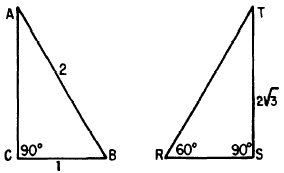Figure 19-11.-Finding parts of 30-60-90 triangles. Answers: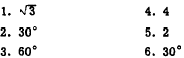THE 45-90 TRIANGLE Figure 19-12 illustrates a triangle in which two angles measure 45 and the third angle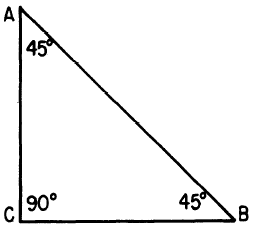Figure 19-12.-A 45-90 triangle. measures 90. Since angles A and B are equal, the sides opposite them are also equal. Therefore, AC equals CB. Suppose that CB is 1 unit long; then AC is also 1 unit long, and the length of AB is calculated as follows: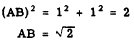Regardless of the size of the triangle, if it has two 45 angles and one 90 angle, its sides are in the ratio 1 to 1 to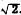. For example, if sides AC and CB are 3 units long, AB is 3units long. Practice problems. Without reference to tables or to the rule of Pythagoras, find the following lengths and angles. in figure 19-13: 1. AB  2. BC  3. Angle B Answers: 1. 22. 2  3. 45Integrated Publishing, Inc. - A (SDVOSB) Service Disabled Veteran Owned Small Business